Chart Pro online
User name (email):

 Recently used

 Forex

 Indices

# Money Flow Index (MFI)

Money flow index (MFI) is technical analysis indicator used to measure the amount of money that "goes" in or out from the financial instrument. It can be used to predict trend reversal.

The basis for calculating MFI is "money flow" (MF). MF is calculated by the formulas:

 Typical Price =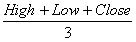Money flow = Typical Price * Volume

MFI compares the ratio of positive and negative money flow. So the final formula for MFI is: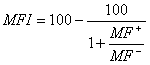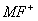- positive money flow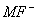- negative money flow

Positive money flow is sum of all money flows which has typical price greater then previous. Negative money flow is sum of all money flows with typical price less then the previous. Usual time period for which the index is calculated is 14 days.

MFI belongs to a group of oscillators. Its values are limited between 0 and 100.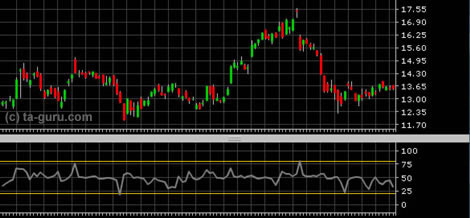Money flow index

Interpretation

Buying and selling signals can be generated depending on the value of indicator. If the value is greater than 80, security is overbought and it should be sold. If the value is less than 20, security is oversold and should be bought. The values of 20 and 80 are most commonly used, but it is possible in practice to use some other values.

Like all other oscillators, MFI can be used for observing divergence. If price falls to new low, but MFI fails to reach new low, downward trend is losing its power and we could expect trend reversal in the near future. Similar goes for upward trend: if money flow index fails to reach new high when price hits new high, upward trend is losing its power.

Example